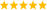# LabVIEW

cancel
Showing results for
Did you mean:

Solved!
Go to solution

## How can I create list of permutations

Hi Everyone,

I need to create a 2 d array which will contain all of the permutations of a 1d input array, specifically, I have a 1 d array of colour boxes and I want to return a 2 d array of colour boxes.  This is too mathematical for me!  Is there a combination of functions that will help me achieve this?  From my days at college I remember something about factorials, but as for coding it...

If anyone has any suggestions I would be very grateful.

Thank you,

Michael.

Message 1 of 8
(6,891 Views)

## Re: How can I create list of permutations

I cannot write you up an example at the moment, because this computer doesn't have LabVIEW, but I can try to explain.

You will need to recursively call a subVI to generate the permutations.

Use the array : [a, b ,c]

- for the first element, it the set can either contain it or not:
[], [a]

- for each of those two arrays, it can either contain the next element or not, so you would insert it

[], [a], [b], [ab]

- for those arrays, it can either contain the next element or not, so you would insert:

[], [a], [b], [ab], [c], [ac], [bc], [abc]

So the subVI would just take the input array, and double it.

For the second half of the new array (the half that was just created), append the most recent element to the end of all the previous elements

Cory K
Message 2 of 8
(6,884 Views)

## Re: How can I create list of permutations

Thanks, I'll give it a go.

Michael

Message 3 of 8
(6,872 Views)

## Re: How can I create list of permutations

Sure, give it a shot.

If you get stuck, I should be able to whip up an example later this afternoon.

Good luck!

Cory K
Message 4 of 8
(6,868 Views)
Solution
Accepted by topic author Michael_78

## Re: How can I create list of permutations

Assuming that all colors in the input array are unique, here's a simple algorithm (LabVIEW 8.5). (see also)The permutation algorithm is from wikipedia:

For every number k, with 0 = k < n!, the following algorithm generates a unique permutation of the initial sequence sj, j = 1, ..., n:

function permutation(k, s) {
for j = 2 to length(s) {
swap s[(k mod j) + 1] with s[j]; // note that our array is indexed starting at 1
k := k / j;        // integer division cuts off the remainder
}
return s;
}
NOTE: Indexing in LabVIEW starts at 0, so the code is changed accordingly.

In LabVIEW 2019 and newer we could accumulate the permutations in a set, eliminating potential duplicates. (Example)LabVIEW Champion. It all comes together inGCentralWhat does "Engineering Redefined" mean??
Message 5 of 8
(6,851 Views)

## Re: How can I create list of permutations

I can not resist the opportunity to implement seven hundred year old algorithms, but do not let me interfere with your fun implementing this.  altenbach and I had some fun a little while back doing this for letters, see this thread.

http://forums.ni.com/t5/LabVIEW/permutations-of-a-6-numerically/td-p/1173677

I implemented Pandit's method to find the next permutation using only the prior one.  This works quite well in situations where you do not want to be carrying around extremely large arrays.  If you want all of the values, just use the second VI.Edit:  Fancy meeting altenbach here.....

Message 6 of 8
(6,846 Views)

## Re: How can I create list of permutations

Thanks Guys, its been fun probing around these block diagrams to see what is going on.  My maths is not what it was 700 years ago (when i was still at school) so I will use the second post, but thanks to you all for the help.

All the best,

Michael.

Message 7 of 8
(6,810 Views)

## Re: How can I create list of permutations

Hi.

Both of the solutions presented here output all possible permutations of the same size as the original array.  Can they be modified, or is there a different way, to find all possible permutations of a smaller size?

For example, for the 6 colors, find all 3-color permutations. We know the number of these permutations is perm(6,3) = 120.

By the way, what I am actually trying to get is the 255,024 permutations you can get selecting 4 elements out of a pool of 24...

Any suggestions?